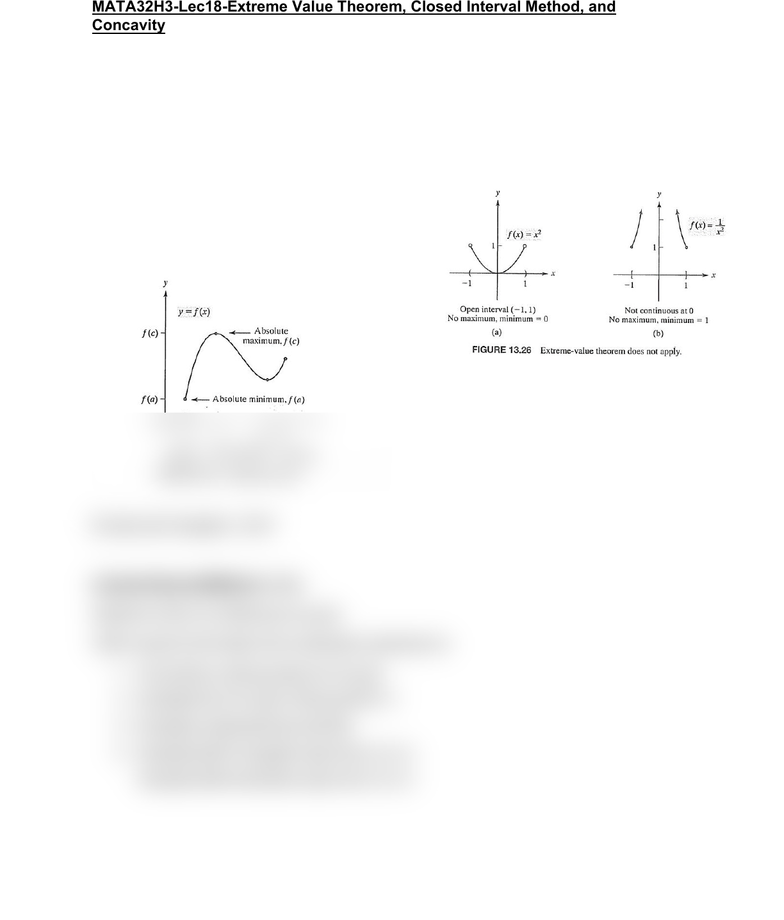Class Notes (1,100,000)
CA (640,000)
UTSC (30,000)
Mathematics (1,000)
MATA32H3 (200)
Lecture 18

# MATA32H3 Lecture Notes - Lecture 18: Arrows Grand Prix InternationalPremium

Department
Mathematics
Course Code
MATA32H3
Professor
Karimian Pour, C.
Lecture
18

This preview shows half of the first page. to view the full 2 pages of the document.MATA32H3-Lec18-Extreme Value Theorem, Closed Interval Method, and
Concavity
Extreme Value Theorem (EVT)
Let y=f(x) be continuous [a,b]
Then f has both an absolute minimum and maximum on [a,b]
Two conditions of using EVT:
1. A closed interval
2. A function continuous on that interval
Ernest and Haussler, 2010
Closed Interval Method (CIM)
Assume y=f(x) is continuous on [a,b]
CIM is used to find where the minimum/ maximum is
1. Find all the critical points of f in [a,b]
2. Evaluate f(c) for each critical point in 1
3. Evaluate endpoints f(a) and f(b)
4. Absolute MAX=longest value from 2 to 3
Absolute MIN=shortest value from 2 to 3